Ex 11.1

Chapter 11 Class 10 Constructions [Deleted]
Serial order wise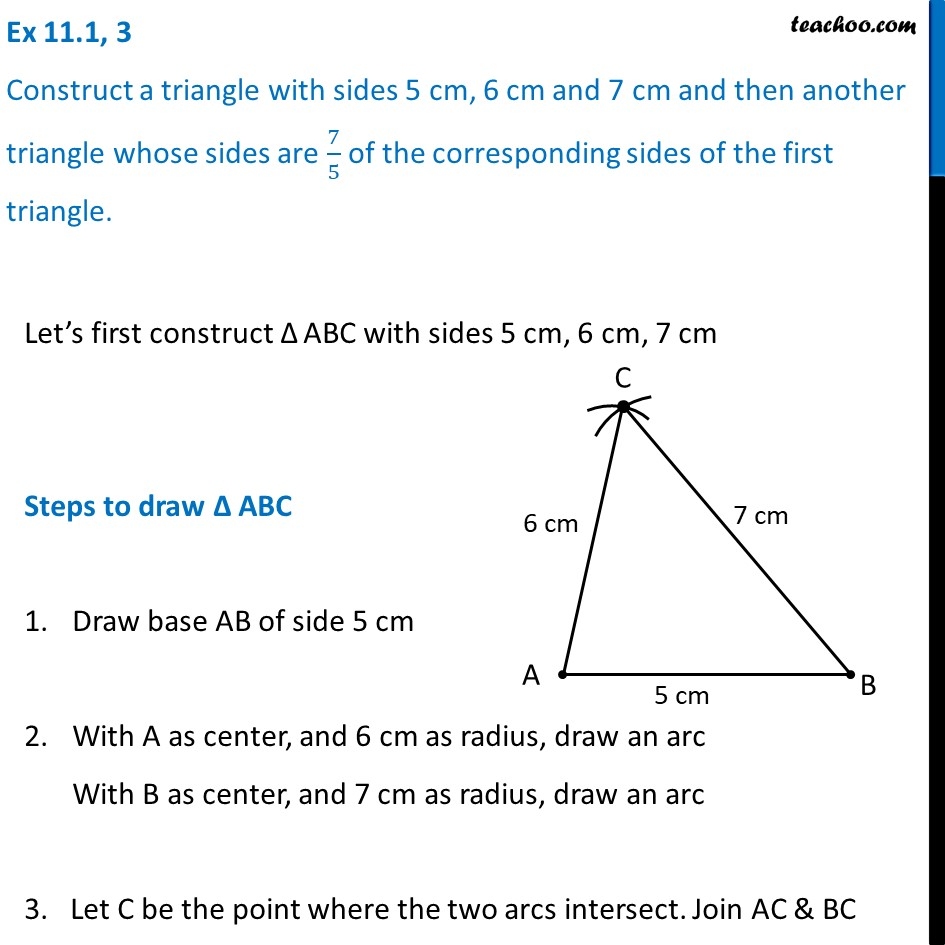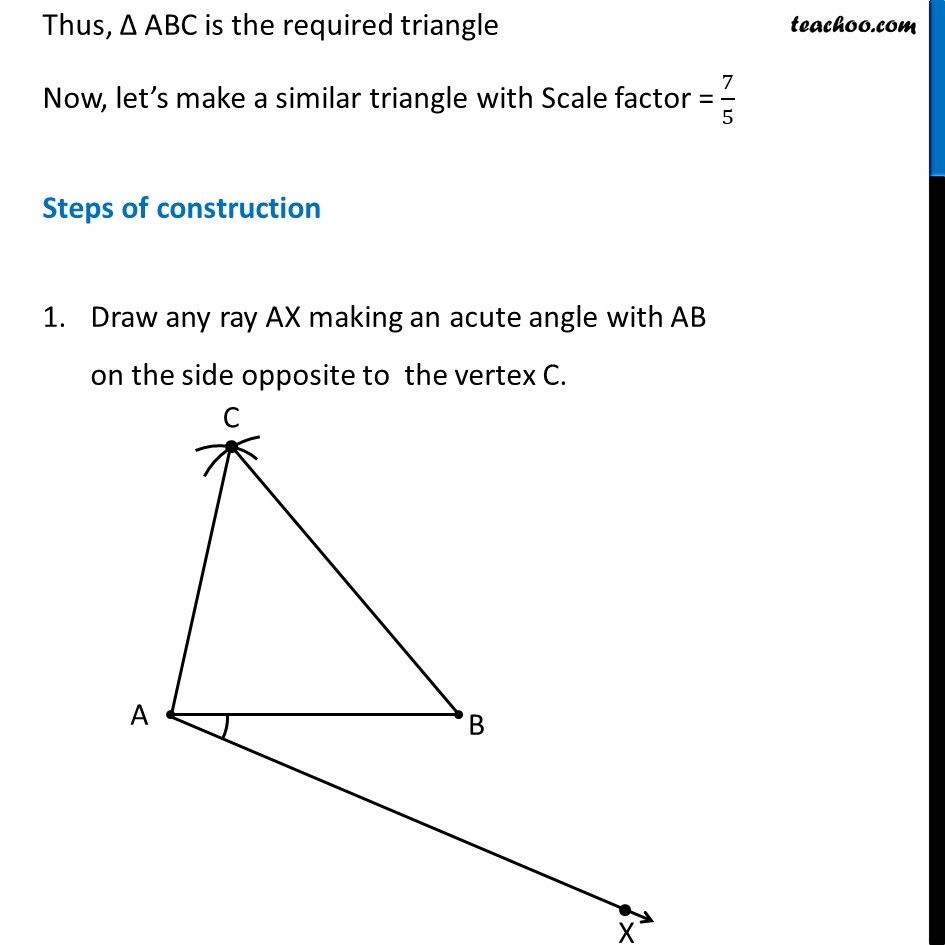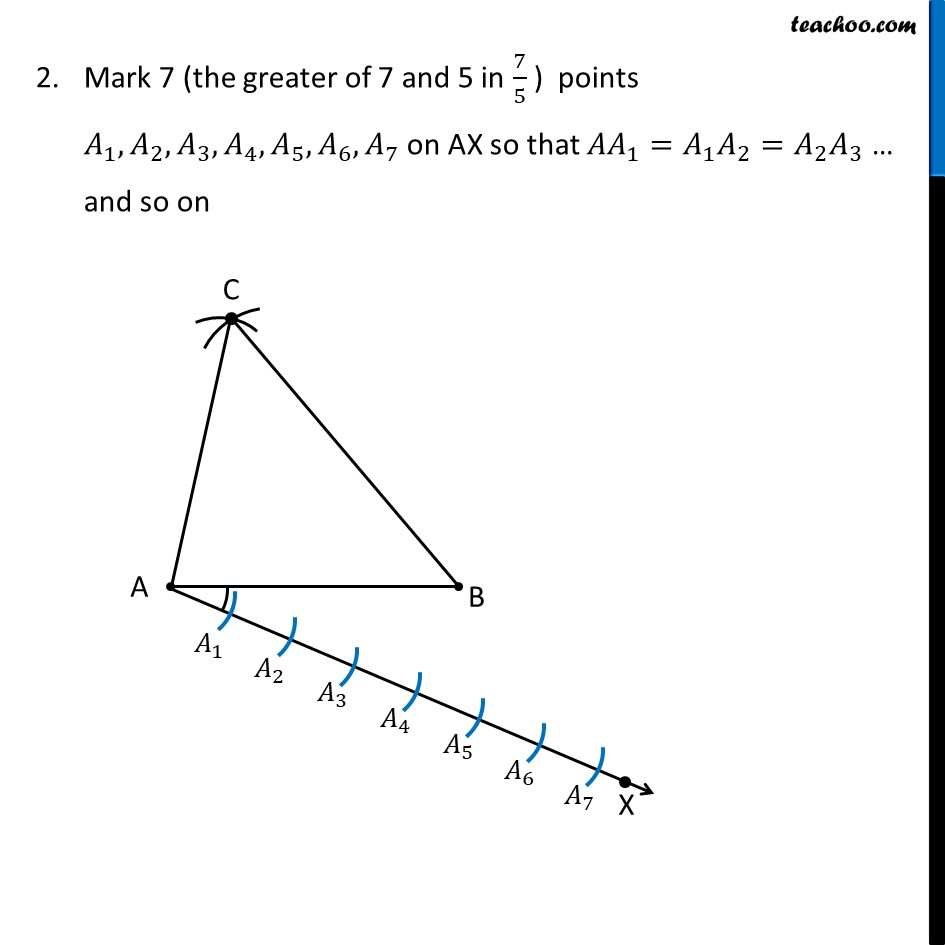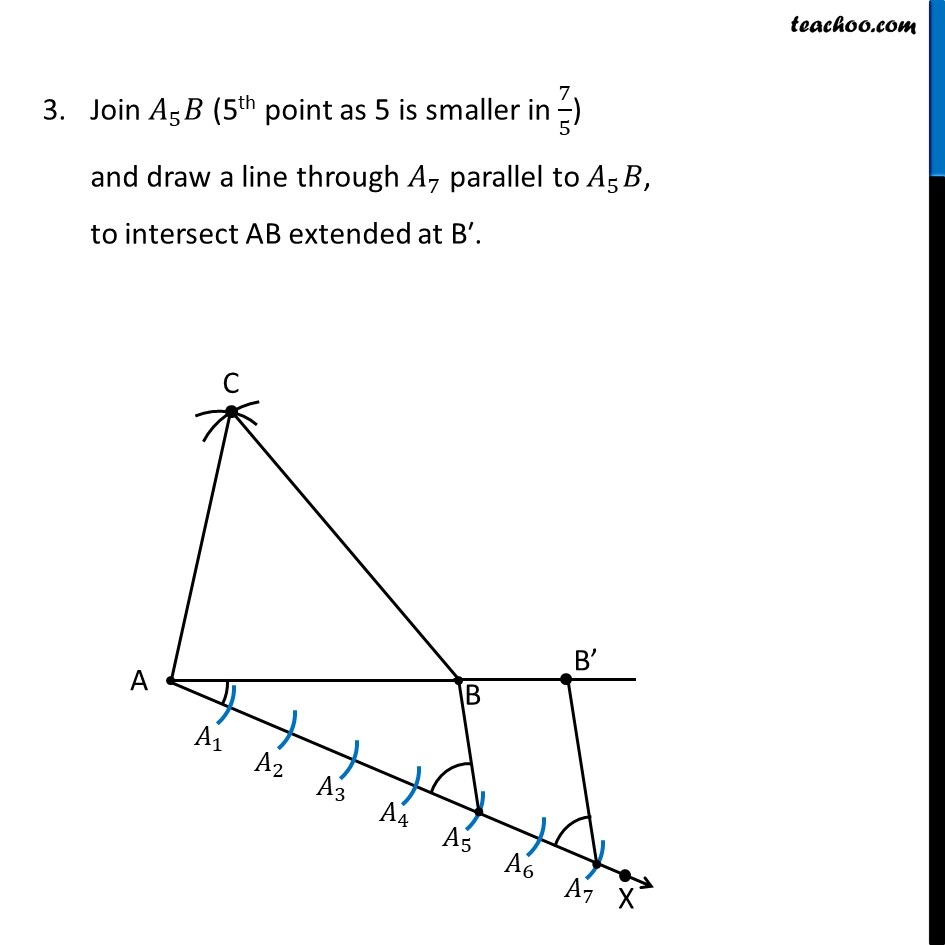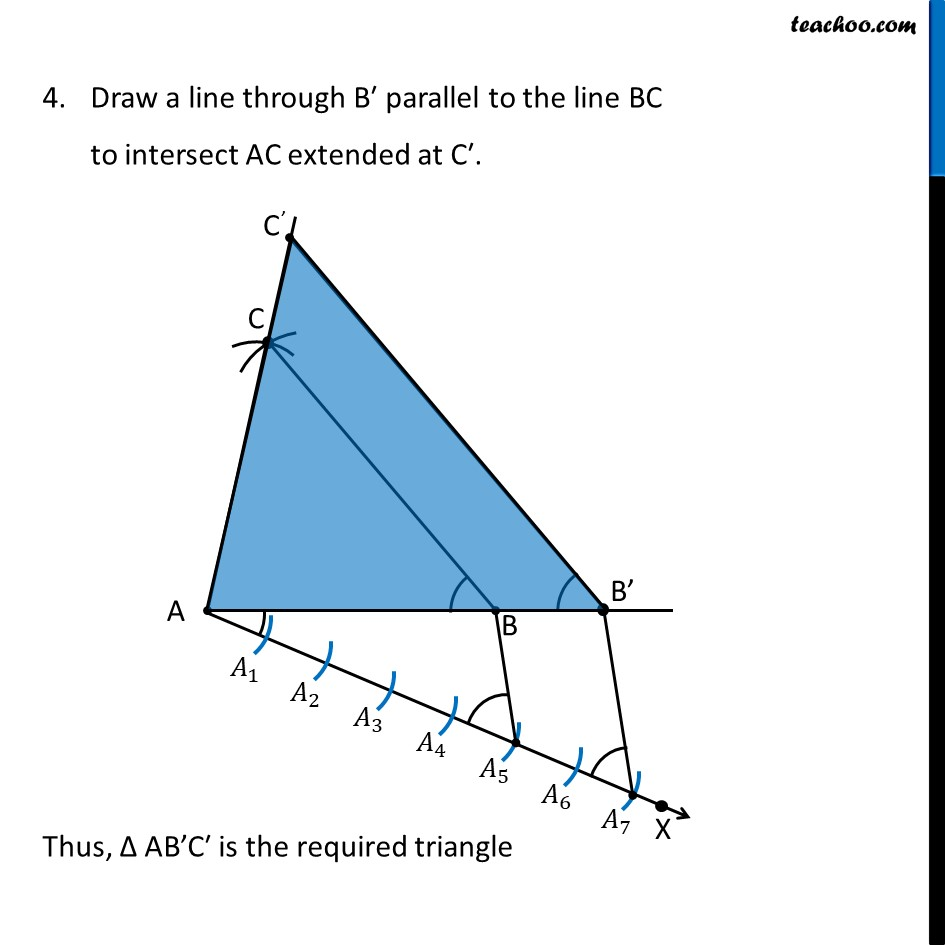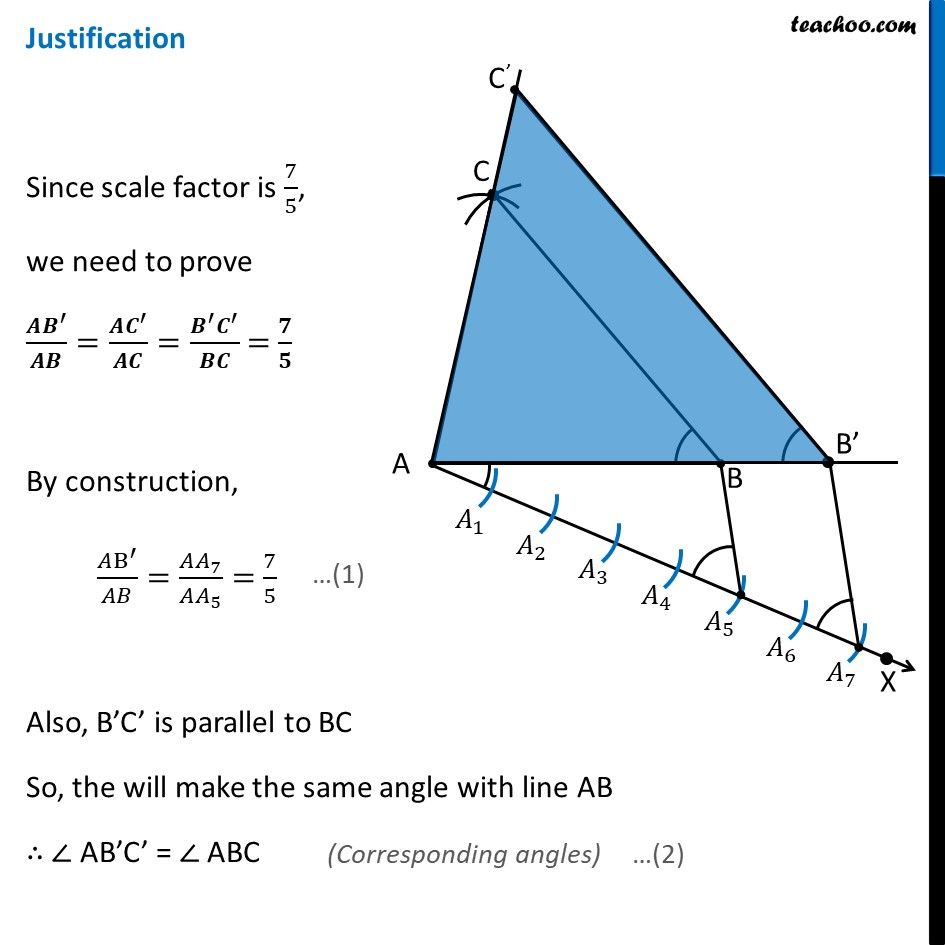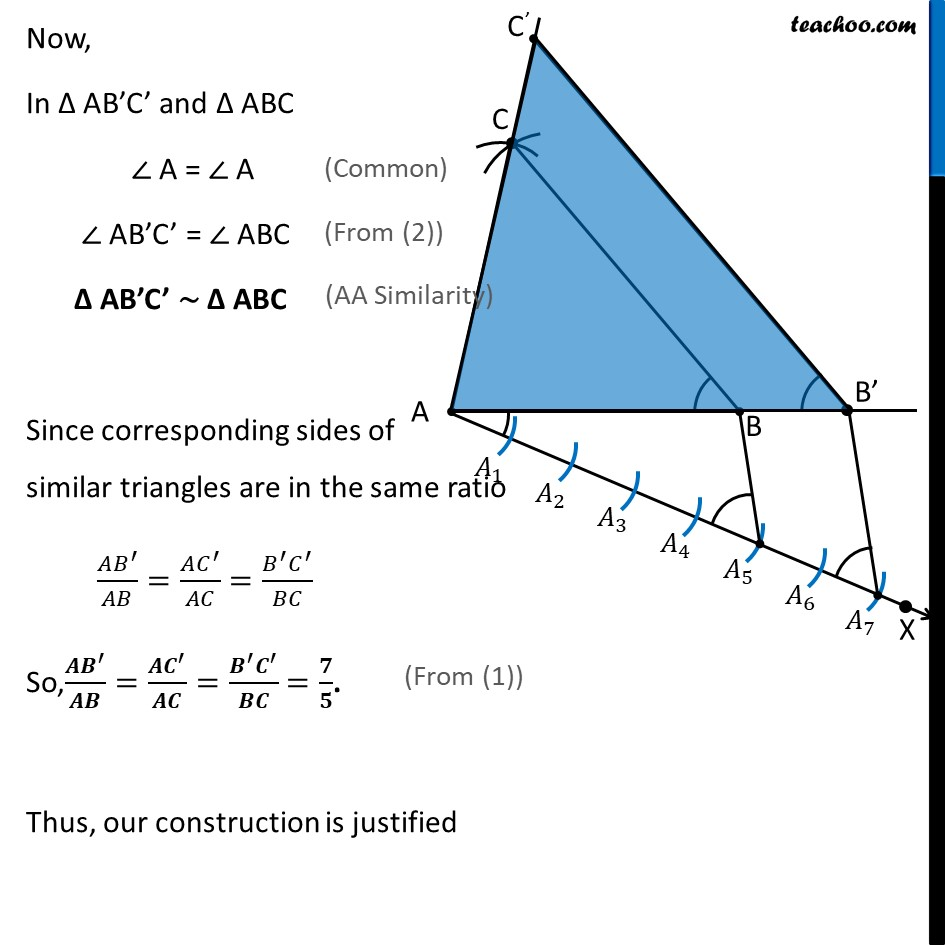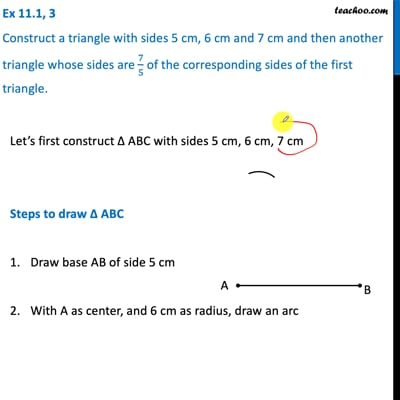This video is only available for Teachoo black users

Solve all your doubts with Teachoo Black (new monthly pack available now!)

### Transcript

Ex 11.1, 3 Construct a triangle with sides 5 cm, 6 cm and 7 cm and then another triangle whose sides are 7/5 of the corresponding sides of the first triangle. Let’s first construct Δ ABC with sides 5 cm, 6 cm, 7 cm Steps to draw Δ ABC Draw base AB of side 5 cm With A as center, and 6 cm as radius, draw an arc With B as center, and 7 cm as radius, draw an arc 3. Let C be the point where the two arcs intersect. Join AC & BC Thus, Δ ABC is the required triangle Now, let’s make a similar triangle with Scale factor = 7/5 Steps of construction Draw any ray AX making an acute angle with AB on the side opposite to the vertex C. Mark 7 (the greater of 7 and 5 in 7/5 ) points 𝐴_1, 𝐴_2, 𝐴_3, 𝐴_4, 𝐴_5, 𝐴_6, 𝐴_7 on AX so that 〖𝐴𝐴〗_1=𝐴_1 𝐴_2=𝐴_2 𝐴_3 … and so on Join 𝐴_5 𝐵 (5th point as 5 is smaller in 7/5) and draw a line through 𝐴_7 parallel to 𝐴_5 𝐵, to intersect AB extended at B′. Draw a line through B′ parallel to the line BC to intersect AC extended at C′. Thus, Δ AB’C′ is the required triangle Justification Since scale factor is 7/5, we need to prove (𝑨𝑩^′)/𝑨𝑩=(𝑨𝑪^′)/𝑨𝑪=(𝑩^′ 𝑪^′)/𝑩𝑪 = 𝟕/𝟓 By construction, (𝐴B^′)/𝐴𝐵=(𝐴𝐴_7)/(𝐴𝐴_5 )= 7/5 Also, B’C’ is parallel to BC So, the will make the same angle with line AB ∴ ∠ AB’C’ = ∠ ABC Now, In Δ AB’C’ and Δ ABC ∠ A = ∠ A ∠ AB’C’ = ∠ ABC Δ AB’C’ ∼ Δ ABC Since corresponding sides of similar triangles are in the same ratio (𝐴𝐵^′)/𝐴𝐵=(𝐴𝐶^′)/𝐴𝐶=(𝐵^′ 𝐶^′)/𝐵𝐶 So,(𝑨𝑩^′)/𝑨𝑩=(𝑨𝑪^′)/𝑨𝑪=(𝑩^′ 𝑪^′)/𝑩𝑪 =𝟕/𝟓. Thus, our construction is justified